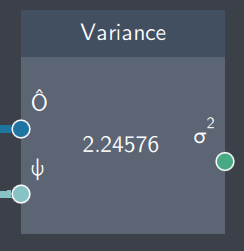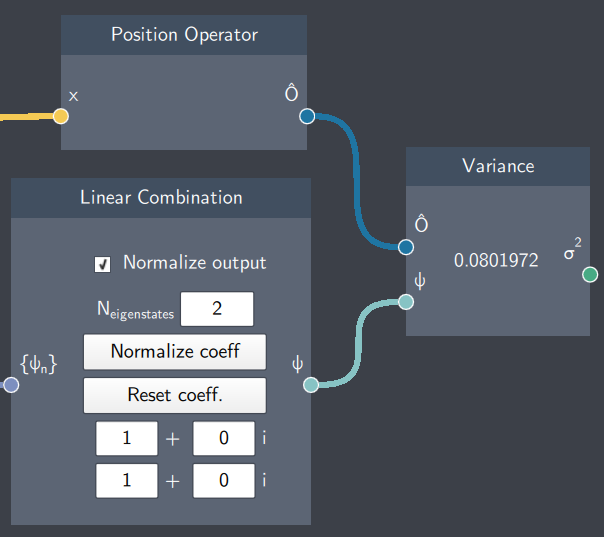# Variance

## Description

This node calculates the variance $\sigma^2= \langle \hat{O}^2 \rangle- \langle \hat{O} \rangle ^2$ of an operator $\hat{O}$. This represents the square of the standard deviation.## Inputs

The node has the following inputs:

• Operator ($\hat{O}$):  Any operator defined in the Operator node such as $\hat{x}$, $\hat{p}$ or $\hat{H}$.
• State ($\psi$) : Any state with regard to which the variance needs to be calculated.

## Output

• Variance ($\sigma^2$): The node outputs the variance of the operator ($\hat{O}$) which is provided in the input.

## Example

In the example below, the variance of the position operator is calculated with regard to a non-stationary state and displayed in the node.